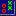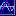# Point and Figure Charts

Point and Figure charts are used to identify support levels, resistance levels and chart patterns. Point & Figure ignores the time factor and concentrates solely on movements in price: a column of X's or O's may take one day or several weeks to complete.

## Point and Figure Calculation

By convention, the first X in a column is plotted one box above the last O in the previous column (and the first O in a column is plotted one box below the highest X).

### Point & Figure Box Size

Each X indicates that price has increased (and each O indicates that price has decreased) by one box. The sensitivity of the chart can be varied by altering the box size. The box size is the minimum price movement recorded and serves to eliminate minor price fluctuations. Larger box sizes are used for charting longer time periods.

### Point & Figure Reversal Amount

A column of X's represents a price advance and a column of O's represents a retracement. A price reversal equal to one box size will result in the formation of a new column. A method of further smoothing out fluctuations is to only record price reversals that exceed a set number of boxes. The number of boxes is called the reversal amount and a new column is only started when price has retraced by that amount.

 X X X X X X O X X O X O X O O X X O X O O X O One Box Reversal

 X X X X X O X X O X O X X O X O O X O Three Box Reversal

The diagram above plots an identical price sequence with different reversal amounts. A 3-box reversal eliminates some of the columns: a new column is not started until price reverses by 3 boxes.

## Point and Figure Setup

To view Point & Figure charts:

• Click View >> Point And Figure on the chart menu; or
• Selecton the toolbar.

#### To create custom Point & Figure chart styles:

1. Select Indicators on the chart menu oron the toolbar.
2. Select Point and Figure on the Indicator Panel.4. Adjust the Box Size on the Indicator Panel
• Log scale box sizes are calculated as a % of the price immediately below. Enter "1" and each box will be calculated as 1% of the preceding price.
• Normal scale box sizes are stepped as the scale changes. Enter "1" and the box size will be set as \$1 for prices over \$100.
See Point & Figure Construction (below) for more details.
5. Choose a price option: High/Low, Closing Price or Typical Price
6. Save your selection > in the right column
7. Close X the Indicator Panel
8. Select Logarithmicor Normalscale on the toolbar or under View on the chart menu.

## Point and Figure Chart Construction

There are numerous methods of constructing Point and Figure charts and supporters still argue over which is the best or correct method. Three main factors affect chart presentation:

1. The data used to construct the charts: either the day's high, low, close, some combination of these or intra-day data;
2. The reversal amount; and
3. Box size.

Most Point and Figure charts use end of day data which is not entirely accurate. Price movements during the day may be incorrectly summarized. For example, an intra-day rally will not be recorded if the end of day close is lower than the previous day. It is therefore advisable to minimize distortion, from end of day data, by using larger box sizes.

### Point & Figure Chart Options

Incredible Charts offer the following Point and Figure options:

1. Boxes are calculated using end of day data
- High or low price for upward movements and downward movements respectively;
- Closing price.
2. Box sizes between 1 and 10 can be selected.
3. Box sizes can either be calculated on a Normal scale or a Logarithmic scale
4. The Reversal amount can be set from 1 to 5 boxes.

## Point & Figure Chart Methods

### De Villiers' Method

The earliest method of Point and Figure charting, introduced by DeVilliers in the 1930's, was a \$1 box and a 1 box reversal - used to track intra-day price movements. It cannot be accurately reproduced on Incredible Charts because the technique mixes X's and O's in the same column and because of the need for intra-day data.

### Classic Point & Figure Method

The Classic method displays X's and O's in separate columns and can be accurately reproduced. Classic Point and Figure chart is set with the following variables:

1. Closing price is used for all calculations;
2. Box sizes are calculated on a normal scale; and
3. The reversal amount is set at 3 boxes.

### Hi/Lo Price

The HiLo method attempts to better identify bullish and bearish sentiment. Price movements are calculated using the day's high when prices are rising and the day's low when prices are falling.

Here is an example of how these charts are plotted using a box size of 1 and a reversal amount of 1. Assume, for this example, that the last box plotted was an X at 20. The boxes are colored according to the day they are plotted (for illustration purposes only).

 Day High Low Price 1 20 17 X 20 2 17 17 O X X 19 3 19 16 O X O X 18 4 19 16 O X O X 17 5 18 15 O O X 16 6 16 15 O 15 7 20 15 14

DAY

1. Compare the High to the last box : Is it at least one box greater? No - Then check for reversal.
Compare the Low to the last box: Is it lower by at least the reversal amount? Yes, 17 is less than (20-1) -
Draw O's from 19 to 17.
2. Compare the Low to the last O : Is it at least one box less? No - Then check for reversal.
Compare the High to the last O: Is it greater by at least the reversal amount? No - Then do nothing.
3. Compare the Low to the last O : Is it at least one box less? Yes - Draw O at 16.
4. Compare the Low to the last O : Is it at least one box less? No - Then check for reversal.
Compare the High to the last O: Is it greater by at least the reversal amount? Yes, 19 is greater than (16+1) - Draw X's from 17 to 19.
5. Compare the High to the last X : Is it at least one box greater? No - Then check for reversal.
Compare the Low to the last X: Is it lower by at least the reversal amount? Yes, 15 is less than (19-1) -
Draw O's from 18 to 15.
6. Compare the Low to the last O : Is it at least one box less? No - Then check for reversal.
Compare the High to the last O: Is it greater by at least the reversal amount? No - Then do nothing.
7. Compare the Low to the last O : Is it at least one box less? No - Then check for reversal.
Compare the High to the last O: Is it greater by at least the reversal amount? Yes, 20 is greater than (15+1) - Draw X's from 16 to 20.

### Typical Price

Typical price is calculated as: (High + Low + Close) / 3.

## Point and Figure Price Scales

### Normal Scale

Originally, point and figure boxes were set at a fixed figure of \$1.00 no matter what the price. This presented some difficulty when plotting very low or very high prices: plotting a stock price of \$3.00 using \$1.00 boxes would not show much movement. The normal scale incorporates several steps in box sizes to allow for this:

 Price Box Size From: Dollars (\$) Cents (c) \$ 0.00 0.1 \$ 0.20 0.2 \$ 0.50 0.5 \$ 1.00 1 \$ 2.00 2 \$ 5.00 5 \$ 10.00 10 \$ 20.00 20 \$ 50.00 50 \$ 100 1.00 100 \$ 200 2.00 \$ 500 5.00 \$ 1000 10.00

The above steps are for a selected box size of 1.

### Logarithmic Scale

Logarithmic scale is useful when plotting substantial price changes, especially over long time periods.

With log scaling, box sizes increase as price increases, enabling you to see the relative changes over a long period of time. Each box size is increased by a set percentage of the box below it.

Box size is set at 1% if you set the box size as 1 on the Indicator Panel (and 10% if you select 10 as the box size).